### 零知识证明 - bellman源码分析

bellman是Zcash团队用Rust语言开发的一个zk-SNARK软件库，实现了Groth16算法。

bellman是Zcash团队用Rust语言开发的一个zk-SNARK软件库，实现了Groth16算法。项目地址：

https://github.com/zcash/librustzcash/tree/master/bellman

## 1. 总体流程## 2. Setup阶段：

Setup阶段最主要的工作是生成CRS数据，相关公式：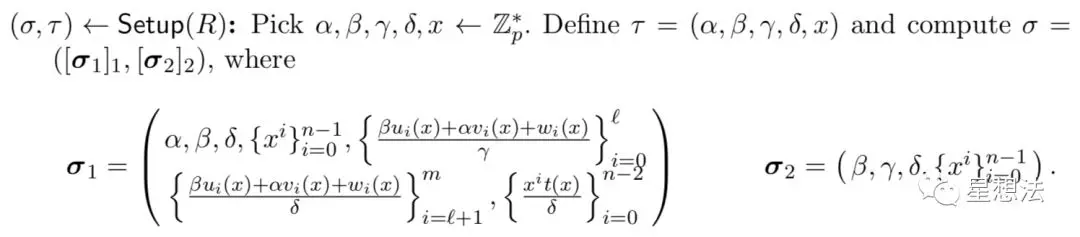### 2.1 参数数据结构

pub struct VerifyingKey<E: Engine> {
pub alpha_g1: E::G1Affine,
pub beta_g1: E::G1Affine,
pub beta_g2: E::G2Affine,
pub gamma_g2: E::G2Affine,
pub delta_g1: E::G1Affine,
pub delta_g2: E::G2Affine,
pub ic: Vec<E::G1Affine>
}

pub struct Parameters<E: Engine> {
pub vk: VerifyingKey<E>,
pub h: Arc<Vec<E::G1Affine>>,
pub l: Arc<Vec<E::G1Affine>>,
pub a: Arc<Vec<E::G1Affine>>,
pub b_g1: Arc<Vec<E::G1Affine>>,
pub b_g2: Arc<Vec<E::G2Affine>>
}

### 2.2 变量类型

Variable类型代表输入数据中的每一个值，分为公开的statement数据和私有的witness数据：

• Input类型：即statement数据

• Aux类型：即witness数据

pub enum Index {
Input(usize),
Aux(usize)
}
pub struct Variable(Index);

### 2.3 ConstraintSystem

ConstraintSystem是一个接口，定义了下面几个函数用于产生不同类型的变量：

• one(): 产生Input类型的变量，索引为0

• alloc(): 产生Aux类型的变量，索引递增

• alloc_input(): 产生Input类型的变量，索引递增

let a = cs.alloc(...)
let b = cs.alloc(...)
let c = cs.alloc_input(...)
cs.enforce(
|| "a*b=c",
|lc| lc + a,
|lc| lc + b,
|lc| lc + c
);

cs.enforce(
|| "a*b=c",
|lc| lc + a + b,
|lc| lc + CS::one(),
|lc| lc + c
);

### 2.4 构建R1CS

Circuit的synthesize()会调用ConstraintSystem的enforce()构建R1CS。 KeypairAssembly是ConstraintSystem的一个实现，R1CS的参数会保存在其成员变量中：

struct KeypairAssembly<E: Engine> {
num_inputs: usize,
num_aux: usize,
num_constraints: usize,
at_inputs: Vec<Vec<(E::Fr, usize)>>,
bt_inputs: Vec<Vec<(E::Fr, usize)>>,
ct_inputs: Vec<Vec<(E::Fr, usize)>>,
at_aux: Vec<Vec<(E::Fr, usize)>>,
bt_aux: Vec<Vec<(E::Fr, usize)>>,
ct_aux: Vec<Vec<(E::Fr, usize)>>
}### 2.5 构建QAP

powers_of_tau.ifft(&worker);
let powers_of_tau = powers_of_tau.into_coeffs();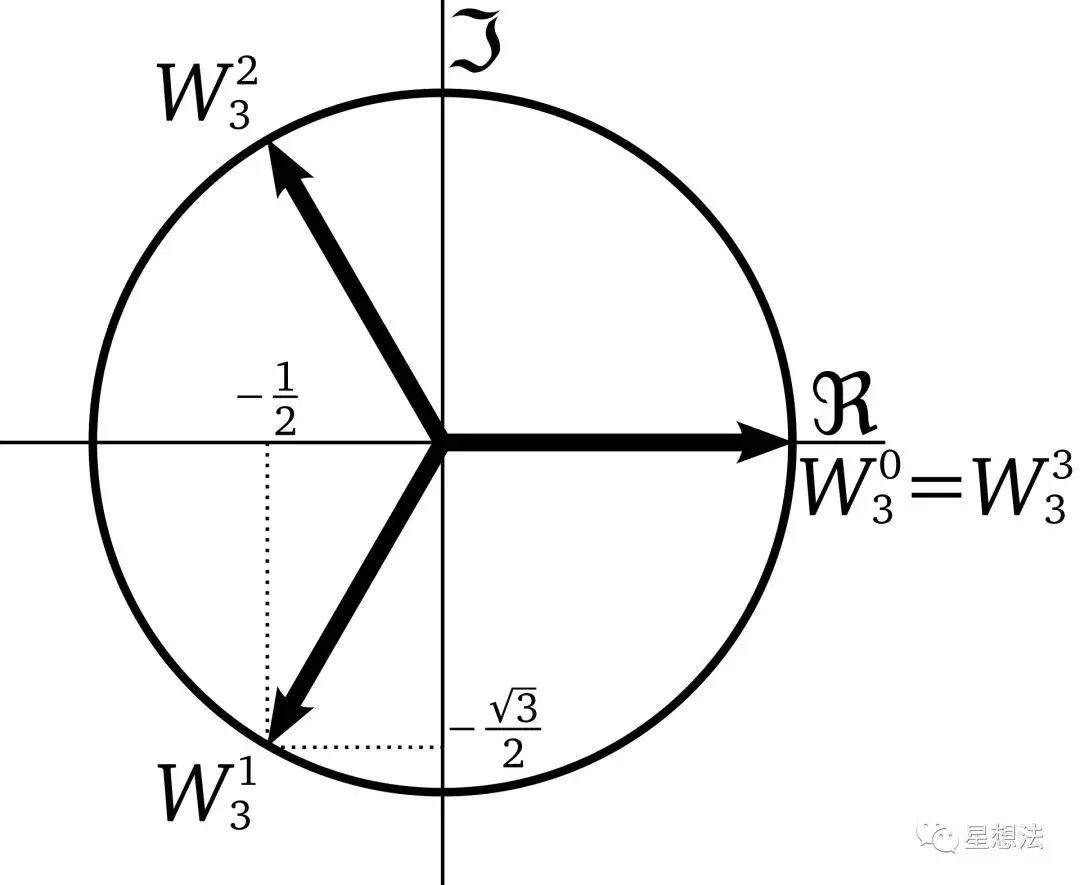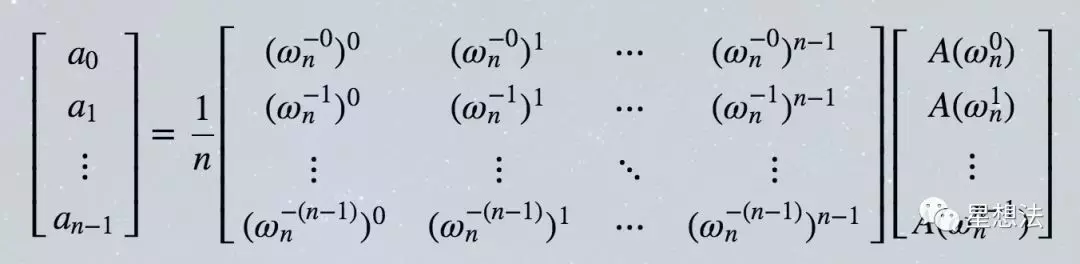### 2.6 准备验证参数pub fn prepare_verifying_key<E: Engine>(
vk: &VerifyingKey<E>
) -> PreparedVerifyingKey<E>
{
let mut gamma = vk.gamma_g2;
gamma.negate();
let mut delta = vk.delta_g2;
delta.negate();

PreparedVerifyingKey {
alpha_g1_beta_g2: E::pairing(vk.alpha_g1, vk.beta_g2),
neg_gamma_g2: gamma.prepare(),
neg_delta_g2: delta.prepare(),
ic: vk.ic.clone()
}
}

fn inverse(&self) -> Option<Self> {
if <Fr as Field>::is_zero(self) {
None
} else {
Some(self.pow(&[(MODULUS_R.0 as u64) - 2]))
}
}

## 3. 创建Proof阶段

Groth16算法生成Proof的公式如下：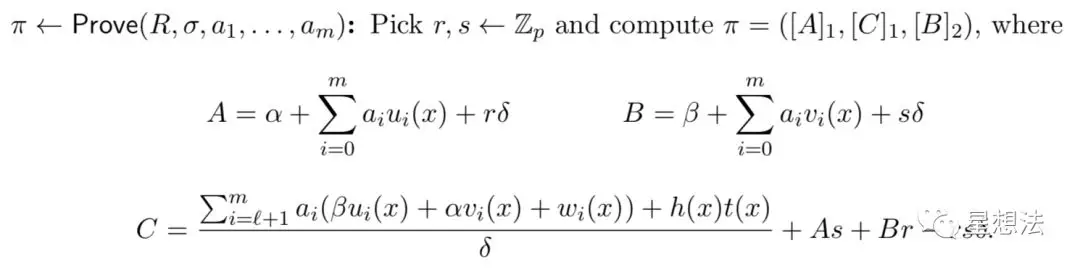pub struct Proof<E: Engine> {
pub a: E::G1Affine,
pub b: E::G2Affine,
pub c: E::G1Affine
}

### 3.1计算$s\cdot A(x),s\cdot B(x),s\cdot C(x)$

struct ProvingAssignment<E: Engine> {
...
a: Vec<Scalar<E>>,
b: Vec<Scalar<E>>,
c: Vec<Scalar<E>>,

input_assignment: Vec<E::Fr>,
aux_assignment: Vec<E::Fr>
}

• alloc()：把witness数据送入aux_assignment中

• alloc_input()：把statement数据送入input_assignment中

• enforce()/eval()：计算出$s\cdot A(x),s\cdot B(x),s\cdot C(x)$，分别放入a、b、c这3个成员变量中

### 3.2 计算h(x)

1.定义$\omega=\sigma^{(r-1)/n}$，则$\omega^n=1$（费马小定理） 2.先通过3次iFFT，计算出a，b，c的多项式系数 3.计算多项式在$\sigma\omega^0,\sigma\omega^1,...,\sigma\omega^{n-1}$这个“偏移集合”上的值 4.再通过3次FFT，获得偏移后的点的集合a'，b'，c' 5.由于$t(\sigma\omega^i)=(\sigma\omega^i)^n-1=\sigma^n-1$，计算h(x)在偏移集合上的点$h(\sigma\omega^i)=\frac{a'_i*b'_i-c'_i}{\sigma^n-1}$ 6.做一次iFFT，计算出偏移后的多项式系数 7.根据尺度变换定理：$f(\sigma x)\leftarrow\rightarrow F(\frac{1}{\sigma}\omega)$，把上一步得到的结果除以σ，就获得了h(x)的多项式系数 根据以上分析，一共需要执行3次FFT，4次iFFT。 bellman基本上就是按照上面的算法来计算的，我们来详细分析一下。

let mut a = EvaluationDomain::from_coeffs(prover.a)?;
let mut b = EvaluationDomain::from_coeffs(prover.b)?;
let mut c = EvaluationDomain::from_coeffs(prover.c)?;

a.ifft(&worker);
a.coset_fft(&worker);
b.ifft(&worker);
b.coset_fft(&worker);
c.ifft(&worker);
c.coset_fft(&worker);

a.mul_assign(&worker, &b);
drop(b);
a.sub_assign(&worker, &c);
drop(c);
a.divide_by_z_on_coset(&worker);

a.icoset_fft(&worker);

multiexp(&worker, params.get_h(a.len())?, FullDensity, a)

## 4. 验证阶段

Groth16算法的proof验证公式如下：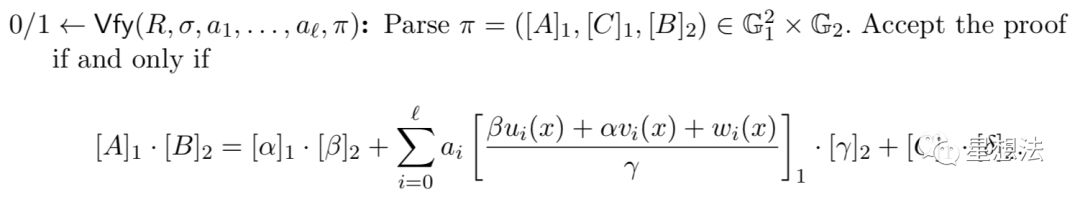let mut acc = pvk.ic.into_projective();

for (i, b) in public_inputs.iter().zip(pvk.ic.iter().skip(1)) {
}

Ok(E::final_exponentiation(
&E::miller_loop([
(&proof.a.prepare(), &proof.b.prepare()),
(&acc.into_affine().prepare(), &pvk.neg_gamma_g2),
(&proof.c.prepare(), &pvk.neg_delta_g2)
].into_iter())
).unwrap() == pvk.alpha_g1_beta_g2)

• 发表于 2019-07-14 23:52
• 阅读 ( 1922 )
• 学分 ( 0 )
• 分类：零知识证明